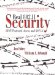# Appendix B. Example Message Modification

 This appendix describes the process needed to modify a WEP-encrypted packet and ensure that the CRC for the packet remains valid, in other words, the bit-flipping attack.Assume that a sender wishes to send a message to a recipient, and that he wishes to use a CRC to detect errors during transmission.Let the message, M(x), be the single ASCII letter N: M(x) = 01001110, and let the CRC generator be G(x) = x3 + x2 + 1, or 1101. To compute the CRC value, M(x) is first multiplied by the degree of G(x), which is 3. Because multiplication in GF(2) is a left shift, we end up with M'(x) = 01001110000. This value is now divided by G(x), and because division in GF(2) is the XOR operation, the result is:` ------------ 1101| 01001110000 1101 1001 1101 1001 1101 1001 1101 1000 1101 1010 1101 1110 1101 110 `The long division results in a remainder of 110, which is now subtracted (subtraction in GF(2) is the XOR operation) from M'(x) to obtain P(x) the message that is sent: P(x)=01001110110.Upon receipt of the message, the recipient divides it with G(x). If the remainder of the division is 0, the message did not contain errors within the precision of the CRC.Real 802.11 Security: Wi-Fi Protected Access and 802.11i
ISBN: 0321136209
EAN: 2147483647
Year: 2005
Pages: 151